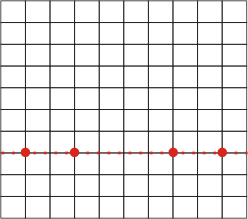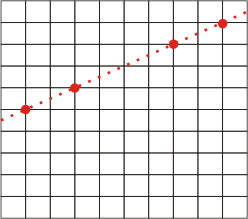### Problem Statement

This problem statement contains images that may not display properly if viewed outside of the applet.

Your backyard is a rectangular grid that measures width x height square meters. You would like to plant treeCount trees using the following rules:

- All trees must be planted at integer coordinates on the grid.

- All trees must lie on the same straight line.

- Each pair of trees must be at least distance meters away from each other.

For example, two (of many) ways in which four trees could be planted on a 10x10 grid if distance is 2 are depicted below:

``

Return the number of distinct ways in which the trees could be planted, modulo 1,000,000,000. Two layouts are considered distinct if there exists a point (x, y) such that one layout contains a tree at (x, y) and the other layout does not.

### Definition

 Class: BackyardTrees Method: countWays Parameters: int, int, int, int Returns: int Method signature: int countWays(int treeCount, int width, int height, int distance) (be sure your method is public)

### Constraints

-treeCount will be between 1 and 50, inclusive.
-width will be between 1 and 500, inclusive.
-height will be between 1 and 500, inclusive.
-distance will be between 1 and 50, inclusive.

### Examples

0)

 `2` `4` `4` `1`
`Returns: 300`
 There are only two trees, and the distance between any two points with integer coordinates is always at least 1. Therefore, you can place the two trees at any two points with integer coordinates. There are 25 such points and this gives you 300 different ways to plant the trees.
1)

 `13` `36` `48` `5`
`Returns: 2`
 The diagonal of the backyard has a length of 60, which is just enough to place 13 trees with a distance of 5 between each adjacent pair. Luckily, these 13 points are at integer coordinates, so you can place the trees along either of the two diagonals while satisfying all the rules.
2)

 `5` `5` `5` `1`
`Returns: 88`
3)

 `50` `49` `49` `1`
`Returns: 102`
 You can place the trees along the rows or the columns of the grid, as well as on the two diagonals.
4)

 `6` `5` `5` `2`
`Returns: 0`
 You can't plant 6 trees on the same line with the necessary distance between them on this grid.
5)

 `10` `55` `75` `5`
`Returns: 490260662`

#### Problem url:

http://www.topcoder.com/stat?c=problem_statement&pm=6620

#### Problem stats url:

http://www.topcoder.com/tc?module=ProblemDetail&rd=10008&pm=6620

_efer_

#### Testers:

PabloGilberto , brett1479 , Olexiy , lovro

#### Problem categories:

Dynamic Programming, Recursion, Simple Math, Simple Search, Iteration